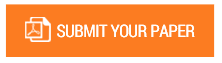Statistical Physical Significance for the Constant Pi other than Circular Geometry

Authors : Dr. Ismail Abbas

Volume/Issue : Volume 6 - 2021, Issue 11 - November

Scribd : https://bit.ly/3ogogAw

Since the time of Archimedes 200 BC and up to the present day, it is still generally accepted that the Greek letter π (Pi) is a mathematical constant approximately equal to 3.142 and is defined as the ratio of the circumference of a circle to its diameter. But it is quite mysterious how it appears in many formulas in most fields of mathematics and physics, even when the geometry of the considered space does not have circular symmetry. In fact, the statistical significance of 2 Pi is that, the energy dissipation time rate (dU / dt) of a system multiplied by its own parameters (absorbent area / volume in unit values) is equal to 2 Pi times its total energy U for any geometry. This article, where we attach statistical physical significance to the constant Pi other than circular geometry, is just a small step on a long road and it is recommended to extend it to different 3D spatial geometries.

#### CALL FOR PAPERS

Paper Submission Last Date
31 - May - 2022